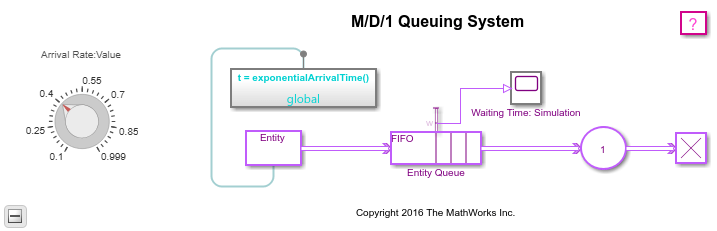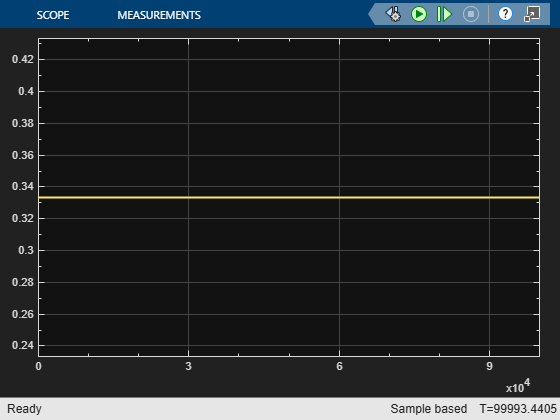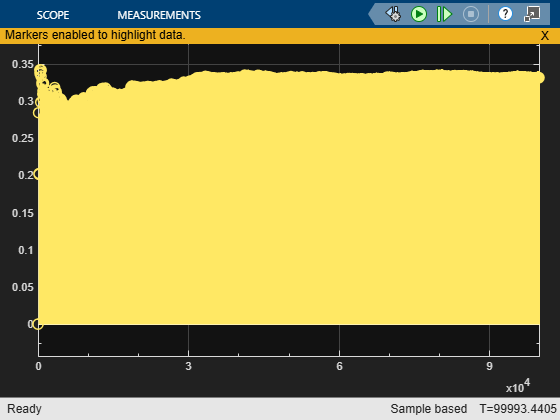Main Content

# M/D/1 Queuing System

### Overview

This example shows how to model a single-queue single-server system that has a Poisson arrival process and a server with constant service time. The queue has an infinite storage capacity. In the notation, the M stands for Markovian; M/D/1 means that the system has a Poisson arrival process, a deterministic service time distribution, and one server.

### Structure

The model includes the components listed below:

• Entity Generator block: Models a Poisson arrival process by generating entities (also known as "customers" in queuing theory).

• Simulink Function exponentialArrivalTime(): Returns data representing the interarrival times for the generated entities. The interarrival time of a Poisson arrival process is an exponential random variable.

• Entity Queue block: Stores entities that have yet to be served in FIFO order

• Entity Server block: Models a server having a constant service time.

This model is similar to the M/M/1 Queuing System model, except that the service time in this model is constant.### Results and Displays

The model includes these visual ways to understand its performance:

• A scope showing the average waiting time of entities (customers) in the queue at over the course of the simulation.### Theoretical Results

According to queuing theory, the mean waiting time in the queue equalswhereis the arrival rate andis the service rate. This duration is half the theoretical mean waiting time in the queue for the M/M/1 queuing system with the same arrival rate and service rate.

### Experimenting with the Model

Move the Arrival Rate Gain knob during the simulation and observe the change in the average waiting time.

### References

 Kleinrock, Leonard, Queueing Systems, Volume I: Theory, New York, Wiley, 1975.

## SupportGet trial now